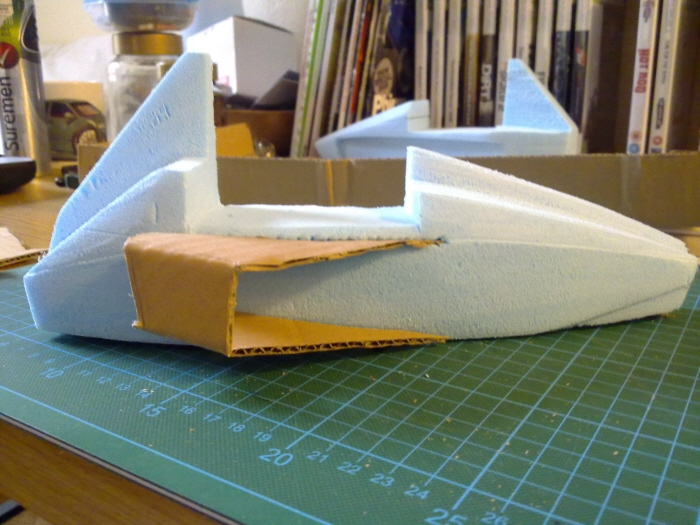Formula Student 2010 - Initial sketch modelsFormula Student 2010 - Initial sketch modelsFormula Student 2010 - Initial sketch modelsFormula Student 2010 - Initial sketch modelsFormula Student 2010 Clay model 1/4 scale - 1/4 scale clay modelFormula Student 2010 Clay model 1/4 scale - 1/4 scale clay modelFormula Student 2010 Clay model 1/4 scale - 1/4 scale clay modelFormula Student 2010 Clay model 1/4 scale - 1/4 scale clay modelFormula Student 2010 Clay model 1/4 scale - 1/4 scale clay modelFormula Student 2010 Clay model 1/4 scale - 1/4 scale clay modelFormula Student 2010 Clay model 1/4 scale - 1/4 scale clay modelFormula student 2010 clay model 1/4 scale - 1/4 scale clay modelFormula student 2010 clay model 1/4 scale - 1/4 scale clay modelFormula student 2010 clay model 1/4 scale - 1/4 scale clay modelFormula student 2010 clay model 1/4 scale - 1/4 scale clay modelFormula student 2010 clay model 1/4 scale - 1/4 scale clay modelFormula student 2010 clay model 1/4 scale - 1/4 scale clay modelFormula student 2010 clay model 1/4 scale - 1/4 scale clay modelFormula Student 2010 clay model 1/4 scale - 1/4 scale clay modelFormula Student 2010 clay model 1/4 scale - 1/4 scale clay modelFormula Student 2010 clay model 1/4 scale - 1/4 scale clay modelFormula Student 2010 clay model 1/4 scale - 1/4 scale clay modelFormula Student 2010 clay model 1/4 scale - 1/4 scale clay modelFormula Student 2010 clay model 1/4 scale - 1/4 scale clay modelFormula Student 2010 clay model 1/4 scale - 1/4 scale clay modelFormula Student 2010 clay model 1/4 scale - 1/4 scale modelFormula Student 2010 clay model 1/4 scale - 1/4 scale modelFormula Student 2010 clay model 1/4 scale - 1/4 scale modelFormula Student 2010 clay model 1/4 scale - 1/4 scale modelFormula Student 2010 clay model 1/4 scale - 1/4 scale modelFormula Student 2010 clay model 1/4 scale - 1/4 scale modelFormula Student 2010 clay model 1/4 scale - 1/4 scale modelFormula student 2010 clay model 1/4 scale - 1/4 scale clay modelFormula student 2010 clay model 1/4 scale - 1/4 scale clay modelFormula student 2010 clay model 1/4 scale - 1/4 scale clay modelFormula student 2010 clay model 1/4 scale - 1/4 scale clay modelFormula student 2010 clay model 1/4 scale - 1/4 scale clay modelFormula student 2010 clay model 1/4 scale - 1/4 scale clay modelFormula Student full size model - Cardboard bodywork to take moulds fromFormula Student full size model - Cardboard bodywork to take moulds fromFormula Student full size model - Cardboard bodywork to take moulds fromFormula Student full size model - Cardboard bodywork to take moulds fromFormula Student full size model - Cardboard bodywork to take moulds fromFormula Student full size model - Cardboard bodywork to take moulds fromFormula Student full size model - Cardboard bodywork to take moulds fromFormula Student 2010 Full size model - cardboard bodyworkFormula Student 2010 Full size model - cardboard bodyworkFormula Student 2010 Full size model - cardboard bodyworkFormula Student 2010 Full size model - cardboard bodyworkFormula Student 2010 Full size model - cardboard bodyworkFormula Student 2010 Full size model - cardboard bodyworkFormula Student 2010 Full size model - cardboard bodyworkFormula Student 2010 full size model - cardboard bodyworkFormula Student 2010 full size model - cardboard bodyworkFormula Student 2010 full size model - cardboard bodywork
Formula Student 2010
Elliot Bond
Conceptual Transport Design Sheffield, United Kingdom